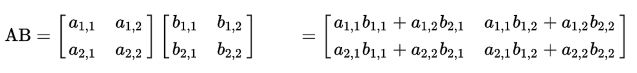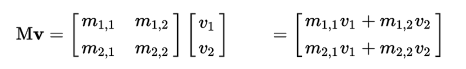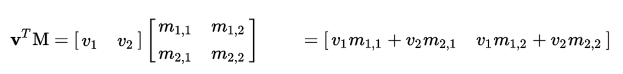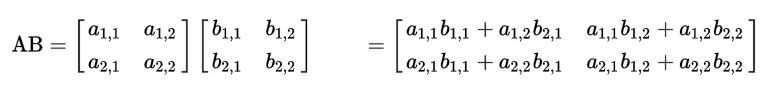## 基本类型

void空类型,即不返回任何值
bool布尔类型 true,false
int带符号的整数 signed integer
float带符号的浮点数 floating scalar
vec2, vec3, vec4n 维浮点数向量 n-component floating point vector
bvec2, bvec3, bvec4n 维布尔向量 Boolean vector
ivec2, ivec3, ivec4n 维整数向量 signed integer vector
mat2, mat3, mat42x2, 3x3, 4x4 浮点数矩阵 float matrix
sampler2D2D 纹理 a 2D texture
samplerCube盒纹理 cube mapped texture

## 向量的分量访问:

GLSL 中向量(vec2,vec3,vec4)往往有特殊的含义，比如可能代表了一个空间坐标（x,y,z,w）或者代表颜色（r,g,b,a），再或者代表一个纹理坐标（s,t,p,q），所以 glsl 提供了更为人性的分量访问方式

`vector.xyzw`其中`xyzw`可以是任意组合
`vector.rgba`其中`rgba`可以是任意组合
`vector.stpq`其中`stpq`可以任意组合

``````vec4 v=vec4(1.0,2.0,3.0,1.0);
float x=v.x; //1.0
float x1=v.r; //1.0
float x2=v; //1.0

vec3 xyz=v.xyz; //vec3(1.0,2.0,3.0)
vec3 mnt=vec(v,v,v); // vec3(1.0,2.0,3.0)
vec3 rgb= v.rgb;  //vec3(1.0,2.0,3.0)

vec2 xyzw = v.xyzw;  //vec4(1.0,2.0,3.0,1.0)
vec2 rgba = v.rgba; //vec4(1.0,2.0,3.0,1.0)``````

## 运算符

1`()`数组:`a*(b+c)`N/A
2`[]\()\.\++\--`数组下标`__[]`,<br/> 方法参数`__fun(arg1,arg2,arg3)`,<br/>属性访问`__a.b__`,<br/>自增/减后缀`__++a a--___`L - R
3`++ \-- \+ \- \!\`自增/减前缀`__++a --a__`,<br/>正负号(一般正号不写)`a`,`-a`,<br/>取反`__!false__`R - L
4`* /`乘除数学运算L - R
5`+ -`加减数学运算L - R
7`< > <= >=`关系运算符L - R
8`== !=`相等性运算符L - R
12`&&`逻辑与L - R
13`^^`逻辑排他或(用处基本等于!=)L - R
14` `逻辑或L - R
15`__? :__`三目运算符L - R
16`= += -= *= /=`赋值与复合赋值L - R
17`,`顺序分配运算L - R
ps 左值与右值:

## 基础类型间的运算

glsl 中,没有隐式类型转换,原则上 glsl 要求任何表达式左右两侧(l-value),(r-value)的类型必须一致 也就是说以下表达式都是错误的:

``````int a =2.0; //错误,r-value为float 而 lvalue 为int.
int a =1.0+2;
float a =2;
float a =2.0+1;
bool a = 0;
vec3 a = vec3(1.0, 2.0, 3.0) * 2;``````

### 1. float 与 int

`float``int`,`int``int`之间可以使直接运算的，但是`float``int`不行，他们需要进行一次显示转换，即要么吧`float`转换成`int:int(1.0)`,要么吧`int`转换成`float:float(1)`,以下表达式都是正确的

``````
int a=int(2.0);
float a=float(2);

int a= int(2.0)*2 + 1;
float a= float92）*6.0+2.3；
``````

### 2 . float 与 vec(向量)mat(矩阵)

vec,mat 这些类型其实有 float 复合而成的，当它们与 float 运算时，其实就是在每一个分量上分别于 float 进行运算，这就是所谓的`逐分量`运算，glsl 里大部分涉及 vec,mat 的运算都是`逐分量`运算，但也并不全是。下文中会讲到的特例

`逐分量`运算是线性的，这就是说 vec 与 float 的运算结果还是 vec.

int 与 vec,mat 之间是不可能运算的，因为 vec 和 mat 中的每个分量都是 float 类型的，无法与 int 类型进行逐分量计算。

``````
vec3 a= vec3(1.0,2.0,3.0);
mat3 m = mat3(1.0);
float s = 10.0;
vec3 b = s * a;   //vec3(10.0,20.0,30.0)
vec3 c = a * s;   //vec3(10.0,20.0,30.0)
mat3 m2 = s * m; //mat3(10.0)
mat3 m3 = m * s;  //mat3(10.0)
``````

### 3. vec(向量)与 vec(向量)：

``````vec3 a= vec3(1.0,2.0,3.0);
vec3 b = vec3(0.1, 0.2, 0.3);
vec3 c = a + b; // = vec3(1.1, 2.2, 3.3)
vec3 d = a * b; // = vec3(0.1, 0.4, 0.9)``````### 4. vec(向量)mat(矩阵)：

``````vec2 v = vec2(10., 20.);
mat2 m = mat2(1., 2.,  3., 4.);
vec2 w = m * v; // = vec2(1. * 10. + 3. * 20., 2. * 10. + 4. * 20.)
...

vec2 v = vec2(10., 20.);
mat2 m = mat2(1., 2.,  3., 4.);
vec2 w = v * m; // = vec2(1. * 10. + 2. * 20., 3. * 10. + 4. * 20.)
``````### 5 . mat(矩阵) 与 mat(矩阵):

``````
mat2 a = mat2(1., 2., 3., 4.);
mat2 b = mat2(10., 20., 30., 40.);
mat2 c = a * b;   //mat2(1.*10.+3.*20.,2.*10.+4.*20.,1.*30.+3.*40.,2.*30.+4.*40.);

mat2 d = a+b;      //mat2(1.+10.,2.+20.,3.+30.,4.+40);``````## 变量限定符

none(默认的可以省略) 本地变量，可读可写，函数的输入参数既是这种类型
const声明变量或函数的参数为只读类型
attribute只能存在于`vertex shader`中一般用于保存顶点或法线数据,他可以在数据缓冲区中读取数据
uniform在运行时`shader`无法改变`uniform`变量，一般用来防止程序传递给`shader`的变换矩阵，材质，光照参数等等
varying主要负责带`vertex``fragment`之间传递变量

### const

const 变量必须在声明时就初始化 `const vec3 v3 = vec3(0.,0.,0.)`

``````
struct light{
vec4 color;
vec3 pos;
//const vec3 posl; //结构中的字段不可用const 修饰符会报错。
}

const light lgt = light(vec4(1.0),vec3(0.0));  //结构变量可以用const修饰
``````

### attribute

attribute 变量是全局只读的，它只能在`vertex shader`中使用，只能与浮点数，向量或矩阵变量组合，一般 attribute 变量用来放置程序传递来的模型顶点，法线，颜色，纹理等书，他可以访问数据缓冲区（还记的`__gl.vertexAttribPointer__`这个函数吧）

`attribute vec4 a_Position;`

### uniform

uniform 变量是全局只读的，整个`shader`执行完毕前其值不会改变，他可以和任意基本类型变量组合，一般我们使用 uniform 变量来防止外部程序传递来的环境数据（如点光源位置，模型的变换矩阵等等）这些数据在运行中显然是不需要被改变的

`uniform vec4 lightPostiton;`

### varying

varying 类型变量是`vetex shader``fragment shader`之间的信使，一般我们在`vertex shader`修改他然后在`fragment shader`使用它，但不能在`fragment shader`修改它

``````
//顶点着色器

varying vec4 v_Color;
void main(){
...
v_Color=vec4(1.,1.,1.,1);

}

//片元着器

...

varying vec4 v_Color;
void main(){
gl_FragColor=v_Color;
}

...
``````

## 函数参数限定符

<none:default>默认使用 in 限定符
in复制到函数中在函数中可读写
out返回时从函数中复制出来
inout复制到函数中并在返回时复制出来

`in`是函数参数的默认限定符，最终真正传入函数的形参其实是实参的一份拷贝，在函数中修改`in`修饰的形参不会影响到实参变量本身

`out`它的作用是向函数外部传递新值，`out`模式下传递进来的参数是`write-only`的（可读不可写）就像是一个“坑位” ，坑位中的值需要函数给他赋予。在函数中修改`out`修饰的形参会影响到实参本身

`inout` inout 下,形参可以被理解为是一个带值的"坑位",及可读也可写,在函数中,修改 inout 修饰的形参会影响到实参本身.

## GLSL 的函数

GLSL 允许在程序的最外部声明函数，函数不能嵌套，不能地推调用，且必须声明返回值类型（无返回值时声明为 void)在其他方面 GLSL 函数与 C 函数非常类似

``````
vec4 getPostiton(){
vec4 v4 = vec4(0.,0.,0.,1.);
return v4;
}

void doubleSize(inout float size){
size = size * 2.0;
}

void main(){
float psize= 10.0;
doubleSize(psize);
gl_Position = getPosition();
gl_PointSize=psize;
}
``````

## 构造函数

glsl 中变量可以在声明的时候初始化，float pSize =10.0 也可以先声明然后等需要的时候在进行赋值。

`````` vec4 color = vec4(0.0, 1.0, 0.0, 1.0);

//一般类型
float pSize = 10.0;
float pSize1;
pSize1=10.0;
...

//复合类型

vec4 color = vec4(0.0,1.0,0.0,1.0);
vec4 color1;

color1= vec4(0.0,1.0,0.0,1.0);
...

//结构

struct light{
float intensity;
vec3 position;
};

light lightVar = =light(3.0,vec3(1.0,2.0,3.0));

//数组

const float c = float(5.0,7.2,1.1);

``````

## 类型转换

glsl 可以使用构造函数进行显示类型转换

``````
bool t = true;
bool f = false;

int a = int (t);  //true转换为1或1.0
int a1 = int (f);  //false转换为0或0.0

float b = float(t);
float b1 = float(f);

bool c = bool(0);  //0或0.0 转换为false
bool c1 = bool(1); //非0转换为true

bool d = bool(0.0);
bool d1 = bool(1.0);

``````

## 精度限定

glsl 在进行光栅化着色的时候，会产生大量的浮点运算，这些运算可能是当前设备所不能承受的，所以 glsl 提供了 3 种浮点数精度，我们可以根据不能的设备来使用合适的精度。

``````
lowp float color;
varying mediump vec3 Coord;
lowp ivec2 foo(lowp mat3);

highp mat4 m;
``````

### 如何确定精度:

``````
uniform highp float h1;
hightp float h2 = 2.3*4.7;//运算过程和结果都是高精度

medium float m;

m=3.7*h1*h2;//运算过程是高精度

h2 = m * h1; //运算过程 是高精度
m = h2 – h1; //运算过程 是高精度
h2 = m + m; //运算过程和结果都 是中等精度
void f(highp float p); // 形参 p 是高精度
f(3.3); //传入的 3.3是高精度
``````

## invariant 关键字:

``````#pragma STDGL invariant(all) //所有输出变量为 invariant
invariant varying texCoord; //varying在传递数据的时候声明为invariant``````

## 限定符的顺序:

1.在一般变量中: invariant > storage > precision

2.在参数中: storage > parameter > precision

``````invariant varying lowp float color; // invariant > storage > precision

void doubleSize(const in lowp float s){ //storage > parameter > precision
float s1=s;
}``````

## 预编译指令:

``````#define #undef #if #ifdef #ifndef #else
#elif #endif #error #pragma #extension #version #line``````

`__LINE__`: 当前源码中的行号.

`__VERSION__` : 一个整数,指示当前的 glsl 版本 比如 100 ps: 100 = v1.00

`GL_ES` : 如果当前是在 OPGL ES 环境中运行则 GL_ES 被设置成 1,一般用来检查当前环境是不是 OPENGL ES.

`GL_FRAGMENT_PRECISION_HIGH`: 如果当前系统 glsl 的片元着色器支持高浮点精度,则设置为 1.一般用于检查着色器精度.

1.如何通过判断系统环境,来选择合适的精度:

``````#ifdef GL_ES //
#ifdef GL_FRAGMENT_PRECISION_HIGH
precision highp float;
#else
precision mediump float;
#endif
#endif``````

2.自定义宏:

``````#define NUM 100
#if NUM==100
#endif``````

## 内置的特殊变量

glsl 程序使用一些特殊的内置变量与硬件进行沟通.他们大致分成两种 一种是 input 类型,他负责向硬件(渲染管线)发送数据. 另一种是 output 类型,负责向程序回传数据,以便编程时需要.

### 在`vertex Shader` 中:

output 类型的内置变量:

highp vec4 `gl_Position`;gl_Position 放置顶点坐标信息vec4
mediump float `gl_PointSize`;gl_PointSize 需要绘制点的大小,(只在 gl.POINTS 模式下有效)float

input 类型的内置变量:

mediump vec4 `gl_FragCoord`;片元在 framebuffer 画面的相对位置vec4
bool `gl_FrontFacing`;标志当前图元是不是正面图元的一部分bool
mediump vec2 `gl_PointCoord;`经过插值计算后的纹理坐标,点的范围是 0.0 到 1.0vec2

output 类型的内置变量:

mediump vec4 `gl_FragColor`;设置当前片点的颜色vec4 RGBA color
mediump vec4 `gl_FragData[n]`设置当前片点的颜色,使用 glDrawBuffers 数据数组vec4 RGBA color

## 内置的常量

1. const mediump int gl_MaxVertexAttribs>=8

`gl_MaxVertexAttribs` 表示在`vertex shader`(顶点着色器)中可用的最大`attributes`数.这个值的大小取决于 OpenGL ES 在某设备上的具体实现, 不过最低不能小于 8 个.

1. const mediump int gl_MaxVertexUniformVectors >= 128

`gl_MaxVertexUniformVectors`表示在`vertex shader`(`顶点着色器)中可用的最大`uniform vectors`数. 这个值的大小取决于 OpenGL ES 在某设备上的具体实现, 不过最低不能小于 128 个.

1. const mediump int gl_MaxVaryingVectors >= 8

`gl_MaxVaryingVectors`表示在`vertex shader`(顶点着色器)中可用的最大`varying vectors`数. 这个值的大小取决于 OpenGL ES 在某设备上的具体实现, 不过最低不能小于 8 个.

1. const mediump int gl_MaxVertexTextureImageUnits >= 0

`gl_MaxVaryingVectors`表示在`vertex shader`(顶点着色器)中可用的最大纹理单元数(贴图). 这个值的大小取决于 OpenGL ES 在某设备上的具体实现, 甚至可以一个都没有(无法获取顶点纹理)

5.const mediump int gl_MaxCombinedTextureImageUnits >= 8

`gl_MaxVaryingVectors` 表示在 `vertex Shader``fragment Shader`总共最多支持多少个纹理单元. 这个值的大小取决于 OpenGL ES 在某设备上的具体实现, 不过最低不能小于 8 个.

1. const mediump int gl_MaxTextureImageUnits >= 8

`gl_MaxVaryingVectors`表示在`fragment Shader`(片元着色器)中能访问的最大纹理单元数,这个值的大小取决于 OpenGL ES 在某设备上的具体实现, 不过最低不能小于 8 个.

1. const mediump int gl_MaxFragmentUniformVectors >= 16

`gl_MaxFragmentUniformVectors` 表示在 `fragment Shader`(片元着色器)中可用的最大`uniform vectors`数,这个值的大小取决于 OpenGL ES 在某设备上的具体实现, 不过最低不能小于 16 个.

1. const mediump int gl_MaxDrawBuffers = 1

`gl_MaxDrawBuffers` 表示可用的 d`rawBuffers`数,在 OpenGL ES 2.0 中这个值为 1, 在将来的版本可能会有所变化.

glsl 中还有一种内置的`uniform`状态变量,`gl_DepthRange`它用来表明全局深度范围.

``````struct gl_DepthRangeParameters {
highp float near; // n
highp float far; // f
highp float diff; // f - n
};
uniform gl_DepthRangeParameters gl_DepthRange;``````

## 流控制

``````for (l = 0; l < numLights; l++)
{
if (!lightExists[l]);
continue;
color += light[l];
}
...

while (i < num)
{
sum += color[i];
i++;
}
...

do{
color += light[lightNum];
lightNum--;
}while (lightNum > 0)

...

if (true)

## 内置函数库

glsl 提供了非常丰富的函数库,供我们使用,这些功能都是非常有用且会经常用到的. 这些函数按功能区分大改可以分成 7 类:

### 通用函数:

T abs(T x)返回 x 的绝对值
T sign(T x)比较 x 与 0 的值,大于,等于,小于 分别返回 1.0 ,0.0,-1.0
T floor(T x)返回<=x 的最大整数
T ceil(T x)返回>=等于 x 的最小整数
T fract(T x)获取 x 的小数部分
T mod(T x, T y) T mod(T x, float y)取 x,y 的余数
T min(T x, T y) T min(T x, float y)取 x,y 的最小值
T max(T x, T y) T max(T x, float y)取 x,y 的最大值
T clamp(T x, T minVal, T maxVal) T clamp(T x, float minVal,float maxVal)min(max(x, minVal), maxVal),返回值被限定在 minVal,maxVal 之间
T mix(T x, T y, T a) T mix(T x, T y, float a)取 x,y 的线性混合,x(1-a)+ya
T step(T edge, T x) T step(float edge, T x)如果 x<edge 返回 0.0 否则返回 1.0
T smoothstep(T edge0, T edge1, T x) T smoothstep(float edge0,float edge1, T x)如果 xedge1 返回 1.0, 否则返回 Hermite 插值

### 角度&三角函数:

T sin(T angle)正弦函数,角度是弧度
T cos(T angle)余弦函数,角度是弧度
T tan(T angle)正切函数,角度是弧度
T asin(T x)反正弦函数,返回值是弧度
T acos(T x)反余弦函数,返回值是弧度
T atan(T y, T x) T atan(T y_over_x)反正切函数,返回值是弧度

### 指数函数:

T pow(T x, T y)返回 x 的 y 次幂 xy
T exp(T x)返回 x 的自然指数幂 ex
T log(T x)返回 x 的自然对数 ln
T exp2(T x)返回 2 的 x 次幂 2x
T log2(T x)返回 2 为底的对数 log2
T sqrt(T x)开根号 √x
T inversesqrt(T x)先开根号,在取倒数,就是 1/√x

### 几何函数:

float length(T x)返回矢量 x 的长度
float distance(T p0, T p1)返回 p0 p1 两点的距离
float dot(T x, T y)返回 x y 的点积
vec3 cross(vec3 x, vec3 y)返回 x y 的叉积
T normalize(T x)对 x 进行归一化,保持向量方向不变但长度变为 1
T faceforward(T N, T I, T Nref)根据 矢量 N 与 Nref 调整法向量
T reflect(T I, T N)返回 I - 2 dot(N,I) N, 结果是入射矢量 I 关于法向量 N 的 镜面反射矢量
T refract(T I, T N, float eta)返回入射矢量 I 关于法向量 N 的折射矢量,折射率为 eta

### 矩阵函数:

mat 可以为任意类型矩阵.

mat matrixCompMult(mat x, mat y)将矩阵 x 和 y 的元素逐分量相乘

### 向量函数:

bvec 指的是由 bool 类型组成的一个向量:

``````vec3 v3= vec3(0.,0.,0.);
vec3 v3_1= vec3(1.,1.,1.);
bvec3 aa= lessThan(v3,v3_1); //bvec3(true,true,true)``````

bvec lessThan(T x, T y)逐分量比较 x < y,将结果写入 bvec 对应位置
bvec lessThanEqual(T x, T y)逐分量比较 x <= y,将结果写入 bvec 对应位置
bvec greaterThan(T x, T y)逐分量比较 x > y,将结果写入 bvec 对应位置
bvec greaterThanEqual(T x, T y)逐分量比较 x >= y,将结果写入 bvec 对应位置
bvec equal(T x, T y) bvec equal(bvec x, bvec y)逐分量比较 x == y,将结果写入 bvec 对应位置

|bvec notEqual(T x, T y)
|bvec notEqual(bvec x, bvec y) |逐分量比较 x!= y,将结果写入 bvec 对应位置|
|bool any(bvec x)| 如果 x 的任意一个分量是 true,则结果为 true|
|bool all(bvec x) |如果 x 的所有分量是 true,则结果为 true|
bvec not(bvec x)| bool 矢量的逐分量取反|

### 纹理查询函数:

``````vec4 texture2DLod(sampler2D sampler, vec2 coord, float lod);
vec4 texture2DProjLod(sampler2D sampler, vec3 coord, float lod);
vec4 texture2DProjLod(sampler2D sampler, vec4 coord, float lod);
vec4 textureCubeLod(samplerCube sampler, vec3 coord, float lod);``````

``````vec4 texture2D(sampler2D sampler, vec2 coord, float bias);
vec4 texture2DProj(sampler2D sampler, vec3 coord, float bias);
vec4 texture2DProj(sampler2D sampler, vec4 coord, float bias);
vec4 textureCube(samplerCube sampler, vec3 coord, float bias);``````

``````vec4 texture2D(sampler2D sampler, vec2 coord);
vec4 texture2DProj(sampler2D sampler, vec3 coord);
vec4 texture2DProj(sampler2D sampler, vec4 coord);
vec4 textureCube(samplerCube sampler, vec3 coord);``````

``````uniform mat4 mvp_matrix; //透视矩阵 * 视图矩阵 * 模型变换矩阵
uniform mat3 normal_matrix; //法线变换矩阵(用于物体变换后法线跟着变换)
uniform vec3 ec_light_dir; //光照方向
attribute vec4 a_vertex; // 顶点坐标
attribute vec3 a_normal; //顶点法线
attribute vec2 a_texcoord; //纹理坐标
varying float v_diffuse; //法线与入射光的夹角
varying vec2 v_texcoord; //2d纹理坐标
void main(void)
{
//归一化法线
vec3 ec_normal = normalize(normal_matrix * a_normal);
//v_diffuse 是法线与光照的夹角.根据向量点乘法则,当两向量长度为1是 乘积即cosθ值
v_diffuse = max(dot(ec_light_dir, ec_normal), 0.0);
v_texcoord = a_texcoord;
gl_Position = mvp_matrix * a_vertex;
}``````

``````precision mediump float;
uniform sampler2D t_reflectance;
uniform vec4 i_ambient;
varying float v_diffuse;
varying vec2 v_texcoord;
void main (void)
{
vec4 color = texture2D(t_reflectance, v_texcoord);
//这里分解开来是 color*vec3(1,1,1)*v_diffuse + color*i_ambient
//色*光*夹角cos + 色*环境光
gl_FragColor = color*(vec4(v_diffuse) + i_ambient);
}
``````